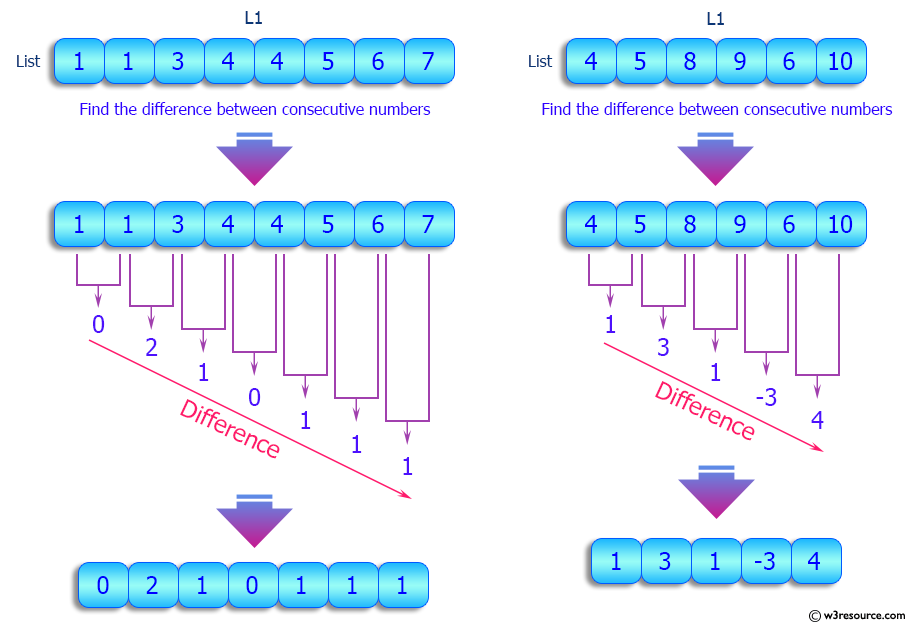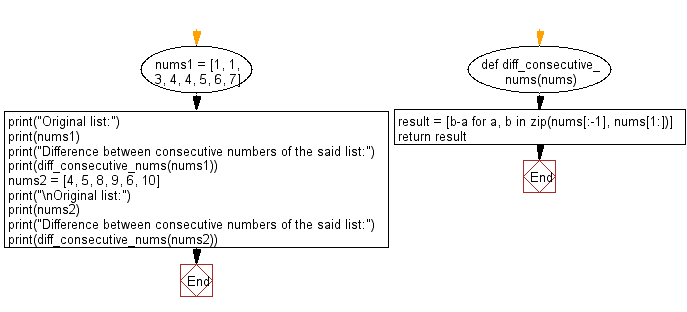﻿ Python: Find the difference between consecutive numbers in a given list - w3resource# Python: Find the difference between consecutive numbers in a given list

## Python List: Exercise - 104 with Solution

Write a Python program to find the difference between consecutive numbers in a given list.

Sample Solution:

Python Code:

``````def diff_consecutive_nums(nums):
result = [b-a for a, b in zip(nums[:-1], nums[1:])]
return result

nums1 = [1, 1, 3, 4, 4, 5, 6, 7]
print("Original list:")
print(nums1)
print("Difference between consecutive numbers of the said list:")
print(diff_consecutive_nums(nums1))

nums2 = [4, 5, 8, 9, 6, 10]
print("\nOriginal list:")
print(nums2)
print("Difference between consecutive numbers of the said list:")
print(diff_consecutive_nums(nums2))
```
```

Sample Output:

```Original list:
[1, 1, 3, 4, 4, 5, 6, 7]
Difference between consecutive numbers of the said list:
[0, 2, 1, 0, 1, 1, 1]

Original list:
[4, 5, 8, 9, 6, 10]
Difference between consecutive numbers of the said list:
[1, 3, 1, -3, 4]
```

Pictorial Presentation:Flowchart:## Visualize Python code execution:

The following tool visualize what the computer is doing step-by-step as it executes the said program:

Python Code Editor:

Have another way to solve this solution? Contribute your code (and comments) through Disqus.

What is the difficulty level of this exercise?

Test your Python skills with w3resource's quiz

﻿

## Python: Tips of the Day

```print(2_000_000)
```2000000